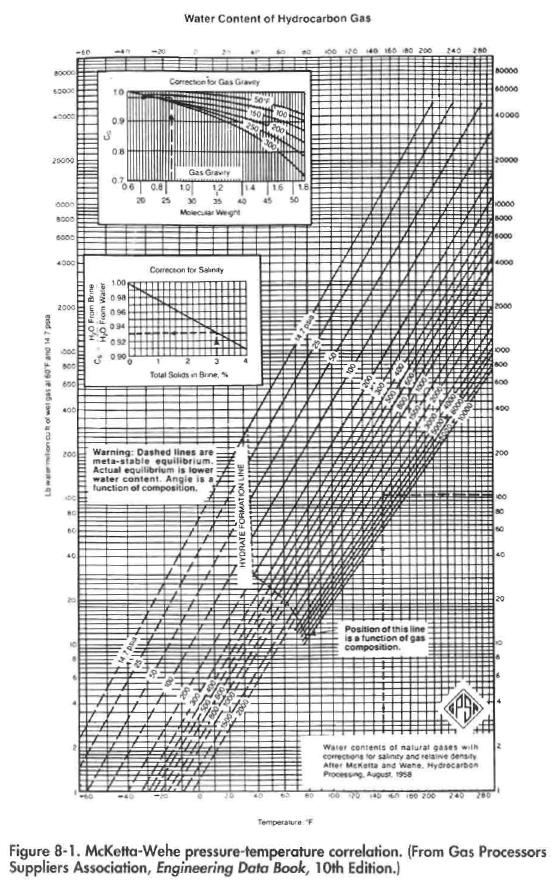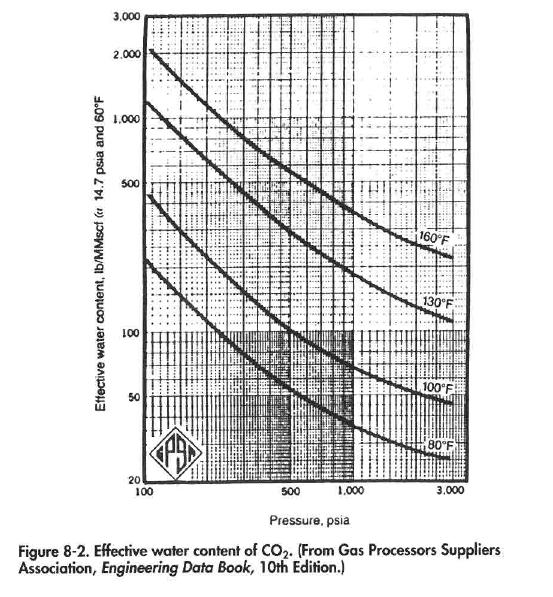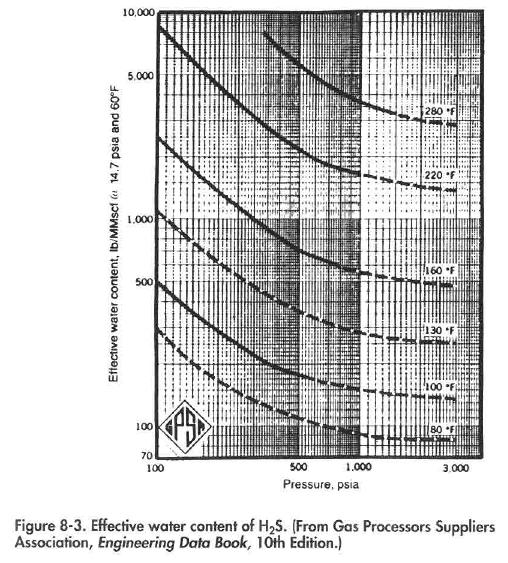# Water Content Determination

The first step in evaluating and/or designing a gas dehydration system is to determine the water content of the gas. The water content of a gas is dependent upon gas composition, temperature, and pressure. For sweet natural gases containing over 70% methane and small amounts of “heavy ends,” the McKetta-Wehe pressure-temperature correlation, as shown in Figure 8-1, may be used. As an example, assume it is desired to determine the water content for a natural gas with a molecular weight of 26 that is in equilibrium with a 3% brine at a pressure of 3,000 psia and a temperature of 150°R From Figure 8-1 at a temperature of 150°F and pressure of 3,000 psia there is 104 Ib of water per MMscf of wet gas. The correction for salinity is 0.93 and for molecular weight is 0.98. Therefore, the total water content is 104 x 0.93 x 0.98 = 94.8 Ib/MMscf.

A correction for acid gas should be made when the gas stream contains more than 5% CO2 and/or H2S. Figures 8-2 and 8-3 may be used to determine the water content of a gas containing less than 40% total concentration of acid gas. As an example, assume the example gas from the previous paragraph contains 15% H2S. The water content of the hydrocarbon gas is 94.8 Ib/MMscf. From Figure 8-3, the water content of H2S is 400 Ib/MMscf. The effective water content of the stream is equal to (0.85X94.8)+ (0.15)(400) or 141 Ib/MMscf.